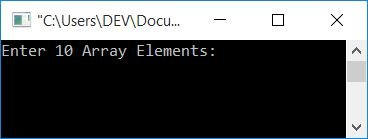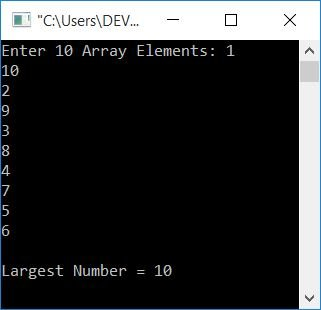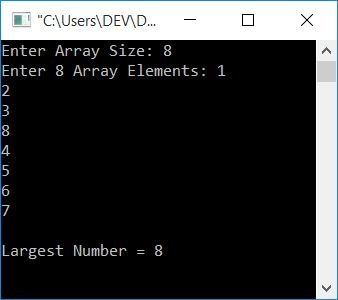# C Program to Find the Largest Number in an Array

In this article, you will learn and get code for finding the largest number from a given list (or array) with and without using a user-defined function.

## Without using a function, find the largest element or number in an array

Let's first create a program without using any functions to find out which is the largest of all.

```#include<stdio.h>
#include<conio.h>
int main()
{
int arr, i, large;
printf("Enter 10 Array Elements: ");
for(i=0; i<10; i++)
scanf("%d", &arr[i]);
i=0;
large = arr[i];
while(i<10)
{
if(large<arr[i])
large = arr[i];
i++;
}
printf("\nLargest Number = %d", large);
getch();
return 0;
}```

This program was built and runs in the Code::Blocks IDE. Here is its sample run:Now supply any 10 numbers and then press ENTER to see the largest one from all the given 10 numbers, as shown below:The program is very simple to understand; the only main logic used is:

1. 0 is initialized to the variable i after getting all the 10 numbers from the user.
2. arr[i] (the first number) is initialized to large.
3. The second statement is created to assume that the first number is the largest one.
4. Compare this number (value in large) to the other nine numbers.
5. If this number (large's value) is less than any of the numbers, then initialize that number to large.
6. After exiting from the while loop, we have a large variable that holds the largest number from the given list.
7. As a result, simply print its value as output.

## Using a Function, find the largest element in an array

Now, let's make the same program with a few extra features. The user can specify the size of the array as an extra feature. And this program is created using a user-defined function named findLargest().

```#include<stdio.h>
#include<conio.h>
int findLargest(int [], int);
int main()
{
int arr, i, large, size;
printf("Enter Array Size: ");
scanf("%d", &size);
printf("Enter %d Array Elements: ", size);
for(i=0; i<size; i++)
scanf("%d", &arr[i]);
large = findLargest(arr, size);
printf("\nLargest Number = %d", large);
getch();
return 0;
}
int findLargest(int arr[], int n)
{
int lrg, i=0;
lrg = arr[i];
while(i<n)
{
if(lrg<arr[i])
lrg = arr[i];
i++;
}
return lrg;
}```

Here is its sample run:#### The same program in different languages

C Quiz

« Previous Program Next Program »